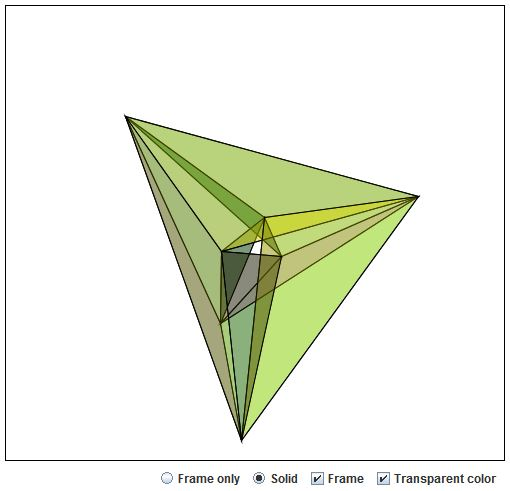# Császár Polyhedron 4

The Császár polyhedron which is topologically equivalent to a torus was discovered in the late 1940s by Ákos Császár [Gardner, p. 139-]. It has 7 vertices, 14 faces, and 21 edges, and is the dual of the Szilassi polyhedron.

### If you are reading this, your browser is not set to run Java applets. Try IE11 or Safari and declare the site https://www.cut-the-knot.org as trusted in the Java setup.What if applet does not run?

Drag the mouse to rotate the prism. Use the right button to remove and put back individual faces.

(Acknowledgement: I have learned most of Java details from the implementation by Meiko Rachimow. I found the geometry of the solid at the software3d online forum.)

... to be continued...

### References

1. M. Gardner, Time Travel and Other Mathematical Bewilderments, W.H.Freeman and Co., NY, 1988.• Right Pentagonal Prism
• Square Pyramid
• Right Triangular Prism
• Twisted Triangular Prism
• Tetrahedron: an Interactive Model
• Octahedron: an Interactive Model
• Cube: an Interactive Model
• Icosahedron: an Interactive Model
• Dodecahedron: an Interactive Model
• Three Pyramids are Better Than Two
• Cube In Octahedron
• Octahedron In Cube
• Octahedron In Tetrahedron
• Tetrahedron In Cube
• Icosahedron In Cube
• Great Stellated Dodecahedron
• Lennes' Polyhedron
• Triangulated Dinosaur
• Volumes of Two Pyramids
• Császár Polyhedron 1
• Császár Polyhedron 4
• Szilassi Polyhedron
• Dissection of a Square Pyramid
•